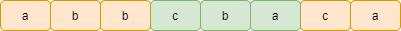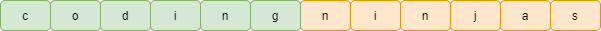# Longest Substring Without Repeating Characters

## Introduction to the problem statement

We are given a string S consisting of English letters, digits and symbols. Our task is to find the length of the largest continuous substring from the given string in which no characters are repeated, i.e. all characters are unique.

Examples:

Input : S= “abbcbaca”

Output : 3Input : S= “codingninjas”

Output : 6## Brute force approach

The idea of this approach is simple: we check all the substrings of the given string and check whether it contains unique characters or repeating characters.

There are a total of n*(n+1)/2 substrings of a string of length n.

Steps:

• We first declare a max_length variable and initialize it with 0.

• Now, we explore all the substrings of the given string using two nested loops:
• Outer loop from (i=0 to n-1) and an inner loop from (j=i to n-1). The idea is to pick a starting character from the outer loop and find all the substrings starting with that character.

• After that, we check whether all the characters in the substring are unique or not with the areDifferent() function.

• If the areDifferent() function returns true, we update the max_length.

• Finally, return the value stored in max_length.

Steps for areDifferent() function

• To check whether the substrings contain unique characters or not, we create a vis array (because the ASCII codes of all the characters and symbols lie in the 0-255 range) and initialize false to every index. It stores the status of all characters, whether it is visited or not.

• We go through each character and check whether it is visited or not. If it is visited, we return false. If no characters are visited twice, we return true.

### Code in C++

``````#include<bits/stdc++.h>
using namespace std;

bool areDifferent(string S, int i, int j)
{
bool vis;

for (int i = 0; i < 256; i++)
vis[i] = false;

for (int k = i; k <= j; k++)
{
if (vis[S[k]] == true)
return false;
vis[S[k]] = true;
}

return true;

}

int longestSubstr(string S)
{
int max_length = 0;

int n = S.length();

for (int i = 0; i < n; i++)
{
for (int j = i; j < n; j++)
{
if (areDifferent(S, i, j))
max_length = max(max_length, j - i + 1);
}
}

return max_length;
}
int main()
{
string S;
cin >> S;
cout << longestSubstr(S) << endl;
return 0;
}``````

Input

``codingninjas``

Output

``6``

### Complexity Analysis

Time Complexity

We are using two nested loops and an areDifferent() function inside it, so the overall complexity becomes O(n2)*O(n) = O(n3)

Space complexity

It is O(1) as we are using a constant size of vis[] array and some variables.

## Sliding window approach

We can further optimise the above solution because, during the loop, if substring S[i to j-1] is already checked, then for substring S[i to j], we only need to check whether S[j] is present in the substring S[i to j-1] or not.

We can use the vis array to check whether it is present in the substring or not.

Steps:

• We first declare a max_length and i variable and initialise them with 0.

• We run an outer loop till i<n to check all the substrings starting from character S[i].

• Inside the loop, we initialize j=i, and both are 0 initially, and we also use a vis array to store the status of characters in the current window [i, j).

• Now, we run an inner loop till j<n && vis[S[j]]==false.

• If S[j] is not present in the current window [i, j), we mark it as visited and keep track of max substring length. Now we move the window to one right by incrementing j.

• If S[j] is already visited, we increment i by 1 and mark the ith character not visited.

When the left end of the string reaches the end, i.e., i=n, we return the value stored in max_length.

### Code in C++

``````#include<bits/stdc++.h>
using namespace std;
int longestSubstr(string S)
{
int max_length = 0;

int n = S.length();

int i = 0;

while (i < n)
{
bool vis = {false};
int j = i;
while (j < n && vis[S[j]] == false)
{
max_length = max(max_length, j - i + 1);
vis[S[j]] = true;
j++;
}
vis[S[i]] = false;
i++;
}

return max_length;
}

int main()
{
string S;
cin >> S;
cout << longestSubstr(S) << endl;
return 0;
}``````

Input

``abbcbaca``

Output

``3``

### Complexity Analysis

Time Complexity

We are using two nested loops, so the time complexity is O(n2)

Space complexity

It is O(1) as we use a constant size 256 of vis[] array and some variables.

## Optimised Sliding window approach

Idea

If the characters in window [i,j] are unique, then the characters in window [i+1, j] will be as well. As a result, there is no need to start with a new window of size 1 or reset j=i in the next iteration of the outer loop.

Steps

• Using the pointers i and j, scan the string from left to right. Maintain an unordered set to keep track of visited characters, as well as the max length variable.

• If S[j] isn't in the set, we insert it and slide the current window by one by incrementing the j pointer. We also update the max_length variable.

• If S[j] already exists in the set, we delete it and move the window to the right by incrementing pointer i.

• If any of the pointers reaches the end of the string, the process will be terminated. We return the value of the max length variable.

### Code in C++

``````#include<bits/stdc++.h>
using namespace std;
int longestSubstr(string S)
{
int max_length = 0;
int n = S.length();
int i = 0, j = 0;
if (n == 0)
return 0;
unordered_set<int> vis;
while (i < n && j < n)
{
if (vis.find(S[j]) == vis.end())
{
vis.insert(S[j]);
j++;
max_length = max(max_length, j - i);
}
else
{
vis.erase(vis.find(S[i]));
i++;
}
}

return max_length;
}

int main()
{
string S;
cin >> S;
cout << longestSubstr(S) << endl;
return 0;
}``````

Input

``codingninjas``

Output

``6``

### Complexity Analysis

Time Complexity

We are using only one while loop, so the time complexity is O(n)

Space complexity

It requires O(n) space for the unordered set.

Q1.What is the sliding window technique?

Ans: As the name implies, this technique involves taking a subset of data from a given array or string and expanding or contracting that subset to satisfy certain conditions, hence the sliding effect.

Q2. Which two pointer technique is used in the quick sort algorithm?

Ans: Most quicksort implementations take advantage of the fact that you can partition by keeping two pointers: one moving in from the left and one from the right.

Q3. What is a binary search algorithm?

Ans: Binary search is a fast algorithm for finding a single item in a sorted list of items. It works by repeatedly dividing the portion of the list that could contain the item in half until you've reduced the number of possible locations to just one.

## Key Takeaways

So, this article discussed the fundamental brute force approach, the sliding window approach, which is better than the brute force approach and optimised sliding window version with linear time complexity of Longest Substring Without Repeating Characters problem and their C++ code.

If you are a beginner, interested in coding and want to learn DSA, you can look for our guided path for DSA, which is free!

In case of any comments or suggestions, feel free to post them in the comments section.